Courses

# CBSE Past Year Paper March - 2015 (Outside Delhi(SET -1)), Marking Scheme, Class 12 Commerce Notes | EduRev

## Commerce : CBSE Past Year Paper March - 2015 (Outside Delhi(SET -1)), Marking Scheme, Class 12 Commerce Notes | EduRev

The document CBSE Past Year Paper March - 2015 (Outside Delhi(SET -1)), Marking Scheme, Class 12 Commerce Notes | EduRev is a part of the Commerce Course Economics Class 12.
All you need of Commerce at this link: Commerce

SECTION - A

(Q1) Define indifference curve.

(1M)

(A1) It is the locus of points representing such bundles of two goods, among which the consumer is indifferent.

(Q2) If due to fall in the price of good X, demand for good Y rises, the two goods are: (Choose the correct alternative)

(1M)

(a) Substitutes

(b) Complements

(c) Not related

(d) Competitive

(A2) (b) Complements.

(Q3) If Marginal Rate of Substitution is increasing throughout, the Indifference Curve will be : (Choose the correct alternative)

(1M)

(a) Downward sloping convex

(b) Downward sloping concave

(c) Downward sloping straight line

(d) Upward sloping convex

(A3) (b) Downward sloping concave.

(Q4) Giving reason comment on the shape of Production Possibilities Curve based on the following schedule :

(3M)

 Good X (units) 0 1 2 3 4 Good Y (units) 30 27 21 12 0

(A4)

 Good X (units) Good Y (units) MRT 0 30 - 1 27 3Y:1X 2 21 6Y:1X 3 12 9Y:1X 4 0 12Y:1X

Since MRT is increasing, the PP curve is downward sloping concave to the origin.

(Diagram not required)

(Q5) What is likely to be the impact of ‘‘Make in India’’ appeal to the foreign investors by the Prime Minister of India, on the production possibilities frontier of India? Explain.

(3M)

OR

What is likely to be the impact of efforts towards reducing unemployment on the production potential of the economy? Explain.

(3M)

(A5) ‘Make in India’ appeal signifies invitation to foreign producers to produce in India. This will lead to increase in resources thus raising production potential of the country. As a result PP curve will shift upwards.      (Diagram not required)

OR

Reducing unemployment has no effect on the production potential of the country. It is because production potential is determined assuming full employment.

Unemployment indicated that the country is operating below potential. Reducing unemployment simply helps in reaching potential.

(Q6) Explain the significance of ‘minus sign’ attached to the measure of price elasticity of demand in case of a normal good, as compared to the ‘plus sign’ attached to the measure of price elasticity of supply.

(3M)

(A6) The measure of price elasticity of demand has a minus sign because there is inverse relation between price and demand of a normal good, while the measure of price elasticity of supply has plus sign because there is direct relation between price and supply of a good.

(Q7) In a perfectly competitive market the buyers treat products of all the firms as homogeneous. Explain the significance of this feature.

(3M)

(A7) This implies that buyers do not differentiate between products of different firms in the industry. As such they are willing to pay only the same price for the products of all the firms. As a result a uniform price prevails in the market.

(Q8) What are the effects of ‘price-floor’ (minimum price ceiling) on the market of a good? Use diagram.

(3M)

Note : The following question is for the Blind Candidates only in lieu of Q. No. 8.

Explain the effects of ‘price-floor’ (minimum price ceiling) on the market of a good.

(A8) When government imposes lower limit on a price that may be charged for a particular good or service, it is called minimum price ceiling e.g. price OP1. At this price the producers are willing to supply P1B or (OQ2) While consumers demand only P1A (=OQ1). Unable to sell all they want to sell, the producers may try to illegally sell below the minimum price. (Answer based on minimum wages is also correct)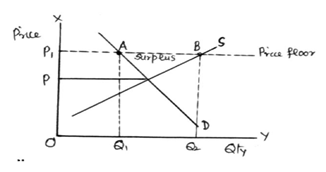For blind Candidates Only :

When government imposes a lower limit on a price that may be charged by the producers of a good or service, it is called price floor.

(1M)

Since this price is above the equilibrium price, at this price producers are willing to supply more but the buyers are willing to buy less. This creates surplus in the market. Due to this producers may adopt illegal ways and sell the product or service at a lower price.

(2M)

(Q9) A consumer spends Rs.1,000 on a good priced at Rs.10 per unit. When its price falls by 20 percent, the consumer spends Rs.800 on the good. Calculate the price elasticity of demand by the Percentage method.

(4M)

A9)

 Price Exp. Demand 10 1000 100 8 800 100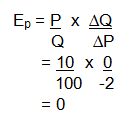(Q10) What is the behaviour of (a) Average Fixed Cost and (b) Average Variable Cost as more and more units of a good are produced?

(4M)

OR

Define Average Revenue. Show that Average Revenue and Price are same.

(A10)

(a) AFC falls continuously as more and more output is produced.

(2M)

(b) AVC falls initially and after a level of output, starts rising as more and more output is produced.

(2M)

OR

Average revenue equals Total Revenue divided by the output produced.

(1M)

TR = P x Q

AR = TR/Q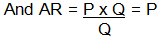(3M)

(Q11) A consumer consumes only two goods X and Y, both priced at Rs.2 per unit. If the consumer chooses a combination of the two goods with Marginal Rate of Substitution equal to 2, is the consumer in equilibrium? Why or why not? What will a rational consumer do in this situation? Explain.

(6M)

OR

A consumer consumes only two goods X and Y whose prices are Rs.5 and Rs.4 respectively. If the consumer chooses a combination of the two goods with marginal utility of X equal to 4 and that of Y equal to 5, is the consumer in equilibrium? Why or why not? What will a rational consumer do in this situation? Use utility analysis.

(A11) Given Px = 2 , Py = 2 and MRS = 2, A consumer is said to be in equilibrium when MRS = Px/Py

Substituting the values we find that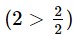i.e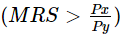Therefore, consumer is not in equilibrium.

(3M)

MRS > means that consumer is willing to pay more for one more unit of X as compared to what the market demands. The consumer will buy more and more of X. As a result MRS will fall due to the Law of Diminishing Marginal Utility. This will continue till MRS = Px/Py and consumer is in equilibrium.

(3M)

(Diagram not required)

OR

Given Px = 5 , Py = 4 and MUx = 4 , MUy = 5 , the consumer will be in equilibrium when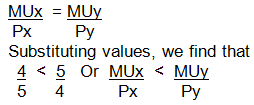(3M)

The consumer is not in equilibrium.

Since per rupee MUx is lower than per rupee MUy , the consumer will buy less of x and more of y. As a result due to Law of Diminishing Marginal Utility, MUx will rise and MUy will fall till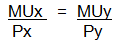(3M)

(Diagram not required)

(Q12) What are the different phases in the Law of Variable Proportions in terms of marginal product? Give reason behind each phase. Use diagram.

(6M)

Note : The following question is for the Blind Candidates only in lieu of Q. No. 12.

Explain with the help of a numerical example different phases in the Law of Variable Proportions.

(A12) The Phases are :

Phase : I MP rises upto A

Phase : II MP falls but is positive i.e. between A and B.

Phase : III MP falls and is negative i.e. after B

(11/2M)

Reasons

Phase I : Initially variable input is too small as compared to the fixed input, As production is increased there is specialization of variable inputs and efficient use of the fixed input leading to rise in productivity of the variable input. As a result MP rises.

(3M)

Phase II : After a level of output a pressure on fixed input leads to fall in productivity of the variable input. MP starts falling but remains positive.

Phase III : The amount of variable input becomes too large in comparison to the fixed input causing decline in total product. MP becomes negative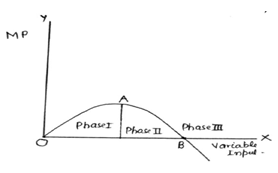(11/2M)

For blind Candidates Only :

 Variable input TP MP (Units) (Unit) (Unit) 1 6 6 2 20 14 3 32 12 4 40 8 5 40 0 6 37 -3

Phases :

(1/2x3M

(1) TP increases at increasing rate upto 2 units.

(2) TP increases at decreasing rate upto 5 units.

(3) TP falls from 6 unit onwards.

Causes :

(3M)

Same as above

(Q13) Explain why will a producer not be in equilibrium if the conditions of equilibrium are not met.

(6M)

(A13) The equilibrium conditions are : (i) MC = MR and (ii) MC > MR after equilibrium

Suppose MC = MR condition is not met. Let MC > MR. In this it will l be profitable for the firm to produce more or less depending upon the relative changes in MC and MR till MC = MR. Similarly, if MC < MR it will also be profitable to produce more till MC = MR.

(3M)

Now Suppose ‘MC > MR after equilibrium condition is not met’ and MC < MR after equilibrium. In this case the firm will not be in equilibrium, because it can increase its profits by producing more.

(3M)

(Diagram not required)

(Q14) Market for a good is in equilibrium. The supply of good ‘‘decreases’’. Explain the chain of effects of this change.

(6M)

(A14) - Given equilibrium, Supply ‘decreases’.

- Price remaining unchanged, excess demand emerges.

- Excess demand leads to competition between buyers causing price to rise.

- Rise in price causes fall (contraction) in demand and rise (expension) in supply.

- Rise in price continues till the market is in equilibrium again at a higher price.

(Diagram not required)

SECTION B

(Q15) What is ‘aggregate demand’ in macroeconomics?

(1M)

(A15) Value of final products the buyers are planning to buy during a given period at a given level of income.

(Q16) If MPC = 1, the value of multiplier is: (Choose the correct alternative)

(1M)

(a) 0

(b) 1

(c) Between 0 and 1

(d) Infinity

(A16) (d) infinity

(Q17) Primary deficit in a government budget is: (Choose the correct alternative)

(1M)

(a) Revenue expenditure – Revenue receipts

(b) Total expenditure – Total receipts

(c) Revenue deficit – Interest payments

(d) Fiscal deficit – Interest payments

(A17) (d) Fiscal deficit Minus interest payment

(Q18) Direct tax is called direct because it is collected directly from: (Choose the correct alternative)

(1M)

(a) The producers on goods produced

(b) The sellers on goods sold

(d) The income earners

(A18) (d) the income earners

(Q19) Other things remaining the same, when in a country the market price of foreign currency falls, national income is likely: (Choose the correct alternative)

(1M)

(a) to rise

(b) to fall

(c) to rise or to fall

(d) to remain unaffected

(A19) (b) to fall

(Q20) If the Real GDP is < 400 and Nominal GDP is < 450, calculate the Price Index (base = 100).

(3M)

(A20)

Real GDP = Nominal GDP x 100                                             (11'2M)

Price Index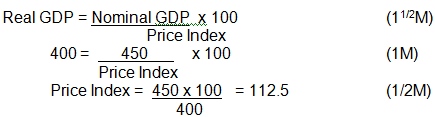(No marks if only the final answer is given)

(Q21) What are fixed and flexible exchange rates ?

(3M)

OR

Explain the meaning of Managed Floating Exchange Rate.

(A21) Fixed Exchange Rate is the exchange rate fixed by the government / central bank and is not influenced by the demand and supply of foreign exchange.

(11/2M)

Flexible exchange rate is the exchange rate determined by the forces of demand and supply of foreign exchange in the market and is influenced by the market forces.

(11/2M)

OR

Managed floating exchange rate is the flexible exchange rate with intervention by the central bank through the market for foreign exchange to reduce fluctuations in the rate. When foreign exchange rate is too high, the central bank starts selling the foreign currency from its reserves. When it is too low central bank starts buying foreign currency in the market.

(3M)

(Q22) Where is ‘borrowings from abroad’ recorded in the Balance of Payments Accounts? Give reasons.

(3M)

(A22) ‘Borrowings from abroad’ is recorded in the ‘capital account’ of BOP account because it increases international liability of the country.

(11/2M)

It is recorded on the credits side because it brings in foreign exchange into the country.

(11/2M)

(Q23) Explain the ‘‘Bankers’ Bank function’’ of the central bank.

(4M)

OR

Explain the ‘‘Bank of Issue function’’ of the central bank.

(A23) As the banker to the banks, the Central Bank holds a part of the cash reserves of commercial banks. From these reserves it lends to commercial banks when they are in need of funds. Central bank also provides cheque clearing and remittance facilities to the commercial banks.

(4M)

OR

The central bank is the sole authority for the issue of currency in the country. It promotes efficiency in the financial system. It leads to uniformity in the issue of currency, and it gives Central Bank control over money supply.

(4M)

(Q24) Currency is issued by the central bank, yet we say that commercial banks create money. Explain. How is this money creation by commercial banks likely to affect the national income? Explain.

(4M)

(A24) Money supply has two components: Currency and demand deposits with commercial banks. Currency is issued by the central bank while deposits are created by commercial banks by lending money to the people. In this way commercial banks also create money.

(2M)

Commercial banks lend money mainly to investors. The rise in investment in the economy leads to rise in national income through the multiplier effect.

(2M)

(Q25) An economy is in equilibrium. Calculate the Investment Expenditure from the following:

(4M)

National Income = 800

Marginal Propensity to Save = 0.3

Autonomous Consumption = 100

(A25) Y = C + MPC(Y) + I                                       (11/2M)

800 = 100 + (1 – 0.3)800 + I                                  (2M)

I = 800 – 100 – 560 = 140                                      (1/2M)

(No marks if only the final answer is given)

(Q26) Giving reason explain how the following should be treated in estimation of national income:

(6M))

(i) Payment of interest by a firm to a bank

(ii) Payment of interest by a bank to an individual

(iii) Payment of interest by an individual to a bank

(A26) (i) Payment of interest by a firm to bank is treated as a factor payment by the firm because the firm borrows money for carrying out production and therefore included in national income.

(2M)

(ii) Payment of interest by bank to an individual is a factor payment because bank borrows for carrying out banking services and therefore included in national income.

(2M)

(iii) Payment of interest by an individual to bank is not included in national income because the individual borrows for consumption and not for production.

(2M)

(No marks if reason is not given)

(Q27) What is ‘deficient demand’? Explain the role of ‘Bank Rate’ in removing it.

(6M)

OR

What is ‘excess demand’? Explain the role of ‘Reverse Repo Rate’ in removing it.

(A27) Deficient Demand: is the amount by which the aggregated demand falls short of aggregate supply at full employment level. It causes fall in price level.

(2M)

Bank Rate: is the rate of interest at which central bank lends to commercial banks for long term. The central bank can reduce deficient demand by lowering Bank Rate. When central bank lowers bank rate. Commercial banks also lower their lending rates. Since borrowing becomes cheaper, people borrow more. This leads to rise in aggregate demand and thus helps in reducing deficient demand.

(4M)

OR

Excess Demand: is the amount by which the aggregated demand exceeds aggregate supply at full employment level. It causes inflation.

(2M)

Reverse Repo Rate: is the rate of interest paid by the central bank on deposits by commercial banks. Central Bank can reduce excess demand by raising the Reverse Repo Rate. When the rate is raised, it encourages the commercial banks to park their funds with the central bank. This reduces lending capacity of the commercial banks. Lending by the commercial banks to public declines leading to fall in aggregate demand

(4M)

(Q28) Explain how the government can use the budgetary policy in reducing inequalities in incomes.

(6M)

(A28) Government can reduce inequalities through its tax and expenditure policy. Government can charge higher rate of tax from higher income groups by imposing higher rate of income tax and higher rate on goods and services purchased by the rich. The money so collected can be spent on the poor in the form of free education, free medical facilities, cheaper housing etc. in order to raise their disposable income.

(Q29) Calculate the ‘National Income’ and ‘Private Income’ :

(6M)

 (Rs. crores) (!) Rent 200 (ii) Net factor income to abroad 10 (iii) National debt interest 15 (iv) Wages and salaries 700 (v) Current transfers from government 10 (vi) Undistributed profits 20 (vii) Corporation tax 30 (viii) Interest 150 (ix) Social security contributions by employers 100 (x)Net domestic product accruing to government 250 (xi) Net current transfers to rest of the world 5 (xii) Dividends 50

(A29)

N.I. = (iv + ix) + i + viii + (vi + vii + xii) – ii                   (11/2M)

= 700 + 100 + 200 + 150 + 20 + 30 + 50 – 10           (1M)

= Rs. 1240 Crore.                                                     (1/2M)

Private Income

= N.I. – x + iii – xi + v                                         (11/2M)

= 1240 – 250 + 15 – 5 + 10                              (1M)

= Rs. 1010 Crore.                                            (1/2M)

(No marks if only the final answer is given)

UNCOMMON QUESTIONS FOR SET 2 & SET 3

(SECTION A)

(Q1) Define Indifference Map.

(1 M)

(A1) A set of indifference curves of a consumer is called indifference map.

(Q2) Distinguish between cooperative and non-cooperative oligopoly.

(3 M)

(A2) When firms in an oligopoly market co-operate with each other in determining price or output or both, it is called co-operative oligopoly. When the firms compete with each other it is called non-co-operative oligopoly.

(Q3) Giving reason comment on the shape of Production Possibilities Curve based on the following schedule :

 Good X (units) 0 1 2 3 4 Good Y (units) 10 9 7 4 0

(A3)

 Good X (units) Good Y (units) MRT 0 10 - 1 9 1Y1X 2 7 2Y1X 3 4 3Y1X (11/2M) 4 0 4Y:1X (11/2M)

Since MRT is increasing, the PP curve will be downward sloping concave to the origin. (Diagram not required)

(Q4) A consumer spends Rs. 400 on a good priced at Rs. 8 per unit. When its price rises by 25 percent, the consumer spends Rs. 500 on the good. Calculate the price elasticity of demand by the Percentage method.

(4 M)

 Price Exp. Demand 8 400 50 10 500 50

(11/2M)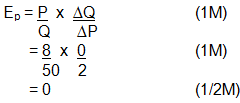(No marks if only thefinal answeris given)

(Q5) A consumer spends Rs.100 on a good priced at Rs.4 per unit. When its price falls by 25 percent, the consumer spends Rs.75 on the good. Calculate the price elasticity of demand by the Percentage method.

(A5)

 Price Exp. Demand 4 100 25 3 75 25 (11/2M)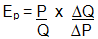(1M)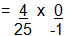(1M)

= 0       (1/2M)

(No marks ff only thefinal answeris given)

(Q6) Market for a good is in equilibrium. Demand for the good “increases”. Explain the chain of effects of this change.

(6 M)

(A6) - Given equilibrium, supply ‘increases.’

- Price remaining unchanged, excess supply emerges.

- Excess supply leads to competition among sellers causing price to fall.

- Fall in price causes rise (expansion) in demand and fall (contraction) in supply.

- These changes continue till the market is in equilibrium again at a lower price.

(Diagram not required)

(SECTION B)

(Q1) If the Real GDP is Rs. 500 and Price Index (base = 100) is 125, calculate the Nominal GDP.

(3 M)

(A1)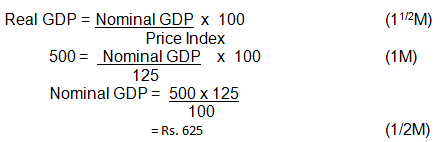(No marks if only the final answer is given)

(Q2) If the Nominal GDP is Rs. 600 and Price Index (base = 100) is 120, calculate the Real GDP.

(3 M)

(A2)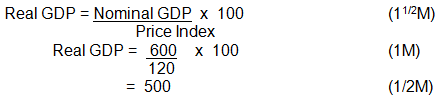(No marks if only the final answer is given)

(Q3) An economy is in equilibrium. Calculate the National Income from the following:

(4M)

Autonomous Consumption  = 120

Marginal Propensity to Save = 0.2

Investment Expenditure = 150

(A3)

Y = C + MPC(Y) + I            (2M)

= 120 + (1 – 0.2)Y + 150      (11/2M)

0.2Y = 270

Y = 1350             (1/2M)

(No marks if only the final answer is given)

(Q4) An economy is in equilibrium. Calculate the Marginal Propensity to Save from the following:

(4M)

National Income = 1000

Autonomous Consumption = 100

Investment = 120

(A4)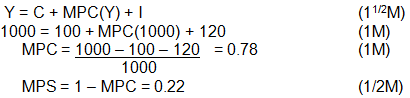(Q5) Calculate ‘Net National Product at Market Price’ and ‘Personal Income’:

(6M)

 (i) Transfer payments by government (Rs. crores) 7 (ii) Government final consumption expenditure 50 (iii) Net imports (-) 10 (iv) Net domestic fixed capital formation 60 (v) Private final consumption expenditure 300 (vi) Private income 280 (vii) Net factor income to abroad (-)5 (viii) Closing stock 8 (ix) Opening stock 8 (x) Depreciation 12 (xi) Corporate tax 60 (xii) Retained earnings of corporations 20

(A5)

NNPmp = v + ii + (iv + viii - ix) – iii - vii       (11/2M)

= 300 + 50 + 60 + 8 – 8 – (-10) – (-5)         (1M)

= Rs.425 Crore.                                        (1/2M)

Personal Income = vi – xi – xii    (11/2M)

= 280 – 60 – 20                         (1M)

= Rs.200 Crore.                         (1/2M)

(No marks if only the final answer is given)

(Q6) Calculate ‘Net Domestic Product at Market Price’ and ‘Gross National Disposable Income’:

(6M)

 (i) Private final consumption expenditure (Rs. crores) 400 (ii) Opening stock 10 (iii) Consumption of fixed capital 25 (iv) Imports 15 (v) Government final consumption expenditure 90 (vi) Net current transfers to rest of the world 5 (vii) Gross domestic fixed capital formation 80 (viii) Closing stock 20 (ix) Exports 10 (x) Net factor income to abroad (-)5

(A6)

NDPmp = i + v + (vii + vii - ii) + (ix – iv) - iiii           (11/2M)

= 400 + 90 + 80 + 20 – 10 + 10 – 15 - 25             (1M)

= Rs.550 Crore.                                                  (1/2M)

GNDI = NDPmp + iii – x - vi                                  (11/2M)

= 550 + 25 – (- 5) – (5)                                          (1M)

= Rs.575 Crore.                                                    (1/2M)

(No marks if only the final answer is given)

Offer running on EduRev: Apply code STAYHOME200 to get INR 200 off on our premium plan EduRev Infinity!

## Economics Class 12

224 videos|237 docs|54 tests

,

,

,

,

,

,

,

,

,

,

,

,

,

,

,

,

,

,

,

,

,

,

,

,

,

,

,

;